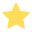Math Time Calculator
Days
dd
Hours
hh
Mins
mm
Seconds
ss
Time
Days
Hours
Mins
Seconds
Initial Time
End Time
--- --- --- ---
--- --- --- ---

# Math Time Calculator

The Math Time Calculator is a tool designed to perform mathematical calculations with time units. Whether the calculation is simple or difficult, it will help you achieve the right result effortlessly with only a few clicks.

Math calculations with time units are always tricky. You need to have into consideration the parts, the whole, and the relation and correspondence between the units in order to solve the problem. A single digit left unaccounted for or wrongly considered can easily lead you to a faulty calculation.

This online time calculator is designed to avoid any of these complications and pitfalls. You will never have to think hard again to add, subtract, multiply or divide time units.

### How to use this Math Time Calculator

Our online math Time Calculator is free and you can use it how many times you want. It is also very intuitive and simple.

Start by filling in the Days, Hours, Minutes, and Seconds fields with your base values. Any field left blank will be considered a zero.

Then use the dropdown menu to select the type of operation you want to perform: addiction, subtraction, multiplication, or division. All that is left to do is to fill in the fields with the values to complete the calculation and press “Calculate”.

The results in days, hours, minutes, and seconds will show up instantly in the results box.